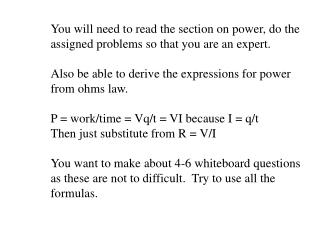# You will need to read the section on power, do the assigned problems so that you are an expert. - PowerPoint PPT PresentationDownload PresentationYou will need to read the section on power, do the assigned problems so that you are an expert.

You will need to read the section on power, do the assigned problems so that you are an expert.
Download Presentation## You will need to read the section on power, do the assigned problems so that you are an expert.

- - - - - - - - - - - - - - - - - - - - - - - - - - - E N D - - - - - - - - - - - - - - - - - - - - - - - - - - -
##### Presentation Transcript

1. You will need to read the section on power, do the assigned problems so that you are an expert. Also be able to derive the expressions for power from ohms law. P = work/time = Vq/t = VI because I = q/t Then just substitute from R = V/I You want to make about 4-6 whiteboard questions as these are not to difficult. Try to use all the formulas.

2. Power • power = work • time • P = IV = I2R = V2 • R • P: Power in W • I: Current in Amps • V: Voltage in Volts • R: Resistance in Ohms Derive all eqn using: V = W/q I = q/t R = V/I Example 1: goes here Example 2: goes here Demo: power supply and heating element, motor and ammeter (I can do these for you?)

3. Whiteboards I think you should do 5 or 6 – try to use all three power equations equally 1 | 2 | 3 | 4 | 5 | 6

4. A heating element draws 2.07 A from a 12.0 volt source. What is the power it consumes? • Given: • P = IV = I2R = V2 • R • P = ?? • V = 12.0 V • I = 2.07 A • R (not given) • Use P = IV • P = 24.84 = 24.8 W W 24.8 W

5. Question goes here? • Given: • P = IV = I2R = V2 • R • R • P = • V = • I = • R = • Use answer

6. Question goes here? • Given: • P = IV = I2R = V2 • R • R • P = • V = • I = • R = • Use answer

7. Question goes here? • Given: • P = IV = I2R = V2 • R • R • P = • V = • I = • R = • Use answer

8. Question goes here? • Given: • P = IV = I2R = V2 • R • R • P = • V = • I = • R = • Use answer

9. Question goes here? • Given: • P = IV = I2R = V2 • R • R • P = • V = • I = • R = • Use answer